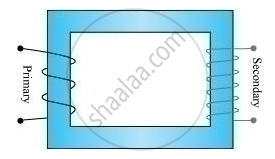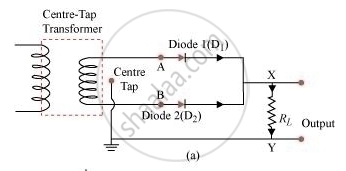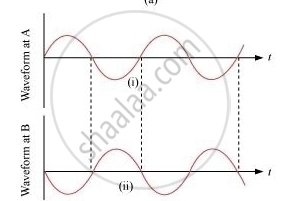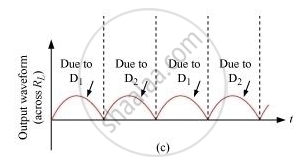Advertisement Remove all ads

# Describe Briefly, with the Help of Labelled Diagram, Working of a Step-up Transformer. a Step-up Transformer Converts a Low Voltage into High Voltage. Does It Not Violate the Principle of - Physics

Advertisement Remove all ads
Advertisement Remove all ads
Advertisement Remove all ads

Describe briefly, with the help of labelled diagram, working of a step-up transformer.

A step-up transformer converts a low voltage into high voltage. Does it not violate the principle of conservation of energy? Explain.

Advertisement Remove all ads

#### SolutionIn a transformer with Ns secondary turns and Npprimary turns, induced emf or voltage Es is:

E_s = -N_s (dphi)/dt

Back emf = Ep =-N_p(dphi)/(dt)

EP = VP

Es = Vs

Thus, Vs = -N_s (dphi)/()dt… (1)

V_p =-N_p (dphi)/dt     ... (2)

Dividing equations (i) and (ii), we obtain

(V_s)/(V_p) = N_s/N_p

If the transformer is 100% efficient, then

i_p v_p = i_s v_s = Power (p)

Thus, combining the above equations,

i_p/i_s = v_s/v_p = N_s/N_p

Or,

V_s = (N_s/N_p)V_p and I_s = (N_p/N_s) I_p

If Ns > Np, then the transformer is said to be step-up transformer because the voltage is stepped up in the secondary coil.

No, the transformer does not violate the principal of conservation of energies. This can be easily observed by the following equation:

V_s I_s =V_pI_p

Power consumed in both the coils is the same as even if the voltage increases or current increases, their product at any instant remains the same.

Concept: Transformers
Is there an error in this question or solution?

#### Video TutorialsVIEW ALL 

Advertisement Remove all ads
Share
Notifications

View all notifications

Forgot password?